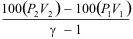Operational Theory

The Ideal Diesel Cycle

 ****JavaScript based drop down DHTML menu generated by NavStudio. (OpenCube Inc. - http://www.opencube.com)****

The indicator diagram is a graph of Pressure against Volume as the piston moves up and down in the cylinder during a cycle of operations.

THE AIR STANDARD DIESEL CYCLE

In thermodynamics, reference is made to the Air Standard Diesel Cycle. It must be made clear that this does not represent what actually happens inside the cylinder of a working diesel engine, but was developed to be able to model engine behaviour and to analyse the effects of certain changes to the cycle without the complications of modelling an actual engine cycle.

Like an indicator diagram it is a graph of Pressure against Volume, but it makes certain assumptions:

The mass of air within the cylinder remains the same: There are no inlet or exhaust valves.

Fuel is not injected and burnt, but heat energy is added at constant pressure.

At the end of the cycle the heat energy is rejected at constant volume.

The compression and expansion of the air is adiabatic (no heat energy is added or lost) and follows the law PVg =C where g for air is 1.4.The graph above is of an ideal diesel cycle for a cylinder of bore 960mm and stroke of 2.5m. Note that the x axis is in m3, not crank angle. However the axis could have also been the stroke of the piston in m, and the diagram would have had the same shape.

Because the diagram is a mathematical model, the temperature, pressure and volume at any point in the cycle can be calculated.

In the members section, download a spreadsheet where you can change the values and see the effect on the work, power and efficiency for engines of different sizes, compression and expansion ratios, changing the values of points 1, 2, 3 and 4.

The area of the diagram using the units of the graph is equal to the work done in the cycle. To calculate this value:

The area between the horizontal line between points 2 and 3 and the x axis represents the work done during the heat addition at constant pressure. This is calculated using the formula P2(V3-V2)100 (kJ). This area is added to the area between the expansion curve (points 3 to 4) and the x axis  which  is calculated using the formula(kJ). The area under the compression curve (points 1 to 2) and the x axis is now calculated using the formula(kJ) and this value is subtracted from the sum of the other two areas. This will give the area enclosed by the diagram.

Note: The figures in the table above use the exact values and are calculated using a spreadsheet, so will differ very slightly from the results if the figures on the graph (which are rounded) are used.

If the work done is divided by the swept volume of the cylinder, then the mean (or average) pressure of the diagram is given. Similarly multiplying the mean pressure by the swept volume will give the work done per cycle.

The Thermal efficiency of the cycle is given by the fomula:orwhere:

r = compression ratio V1/V2

r = ratio of the burning period volumes V3/V2 (= T3/T2)

g = adiabatic index of compression/expansion (1.4 for air)

IN THE MEMBERS SECTION: